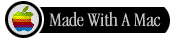## Learning Prolog via Examples - How does it work?

 Part of INTERACTIVE PROLOG GUIDE Prev TOC Next

In this lesson I try to collect some features of Prolog language which I have found to be difficult to understand by students accustomed to procedural style of programming.

is
"is" is a build-in arithmetic evaluator in Prolog. "X is E" requires X to be free variable and E to be arithmetic expression that is possible to evaluate. E can contain variables but these variables has to be bound to numbers, e.g., "X=5, Y is 2*X" is correct Prolog goal. Note, that we can use unification to bind variables but do not interchange unification and evaluation. Also, do not use "is" in the same way as assigment in procedural languages! Following examples shows wrong usage of "is":
```?-X is Y+2.              % arithmetic expression cannot be evaluated
?-1 is 3-2.              % first argument of "is" is not free variable
?-X is 1+2, X is 2*X.    % first argument of second "is" is not free
?-H=5,T=[], L is [H|T].  % expression [H|T] is not arithmetic```

unification
Unification is used to bind variables as well as to compare data structures. Unification does not evaluate expressions.
```?-X=f(Y).         % returns X/f(Y)
?-f(g(Y))=f(X).   % returns X/g(Y)
?-X=1+2.          % returns X/1+2
?-3=1+2.          % fails (3 is syntactically different from 1+2)```

backtracking
Backtracking is a powerfull feature of Prolog that simplifies development of many programs. It enables the program to use other alternative if the previous alternative fails. Thus, programming of generate and test algorithms is natural in Prolog. Also, it is usually possible to find one solution as well as all solutions using the same program.

declarative character
Declarative character of many Prolog programs enables one to use the same procedure in different ways. Note, that it is not distinguished whether the argument of the procedure is input or output in Prolog. Thus, it is possible to use one argument as input in one call and use the same argument as output in other call. See following example:
```member(X,[X|T]).
member(X,[_|T]):-member(X,T).

?-member(1,[1,2,3]).  % usage as a test
?-member(X,[1,2,3]).  % usage as member generator (returns successively X=1, X=2, X=3)
?-member(1,L).        % usage as list generator (returns L=[1|_], L=[_,1|_], L=[_,_,1|_] etc.)
?-member(X,L).        % generator (returns L=[X|_], L=[_,X|_], L=[_,_,X|_] etc.)```

operators
Operators simplify entry of Prolog programs. They are only used to define syntactic conventions of program and data entry. The definition of operators helps Prolog to undersatnd expression like 1+2+3*4 which is translated into notation +(+(1,2),*(3,4)).

cut
Cut is a feature of Prolog (not logic programming) that is used to cut alterantive branches of compuation and, thus, these branches are not explored by backtracking. Cut can improve efficiency of Prolog programs, however, it also changes the clear operational behaviour of programs. Use "cut" carefully as the programs containing cut are usually harder to read. The following example explains the behaviour of cut.
```?-member(Y,[[1,2],[3,4]]),member(X,Y).     % returns X=1,X=2,X=3,X=4 successively
?-member(Y,[[1,2],[3,4]]),member(X,Y),!.   % returns X=1 only
?-member(Y,[[1,2],[3,4]]),!,member(X,Y).   % returns X=1, X=2 succesively
?-!,member(Y,[[1,2],[3,4]]),member(X,Y).   % returns X=1,X=2,X=3,X=4 successively```

negation
Because of complexity reasons, Prolog does not contain full logic negation. Instead of it, Prolog uses negation as failure which is based on Closed World Assumption. The operational behaviour of this type of negation can be expressed by the following program:
```not P:-P,!,fail.
not P.```
Negation in Prolog can be safely used only as a test. Assure that all variables in negated goal are bound, otherwise you can get "strange" results as following examples show:
```p(a).
p(b).
q(c).

?-not p(X), q(X).   % fails
?-q(X), not p(X).   % succeeds with X=c```

If you do not understand how does something work, then try some simple examples.

 Part of INTERACTIVE PROLOG GUIDE Prev TOC NextLast update 26nd December 1997
Designed and maintained by Roman Bartak